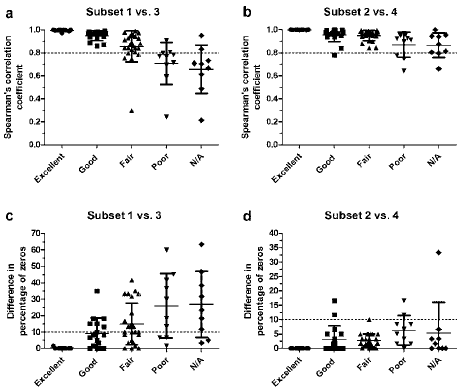Figure 4: Correlation and difference in percentage zeros seen with comparisons over time in the subjective groups. (a and b) Spearman’s correlation coefficient (rs) was calculated from plots of metabolite concentrations determined from analysis done at two different time points.(a) Subsets 1 vs. 3, (b) Subsets 2 vs. 4. (c and d) Difference in percentage zeros between the two time points. (c) Subsets 1 vs. 3, (d) Subsets 2 vs. 4. The grey shaded area represents metabolites that are identified as difficult to quantify in all graphs: (a) and (b) rs<0.8; (c) and (d) difference in percentage of zeros >10. The metabolites rated subjectively in Table 1 retain their grouping here. N/A is Not Analyzed. Error bars represent standard deviation of the values.